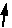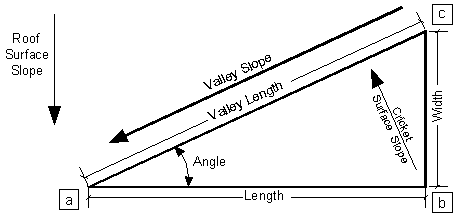Valley Slope Calculators
 Calculator 1   solves for valley slope, rise, angle and ratio based on surface slope, length and width.
 Roof Surface Slope Length(a) to (b) Width(b) to (c) Resulting Valley Slope(c) to (a) Valley Length(a) to (c) Rise (b) to (c)(based on roof surface slope) Length to Width Ratio Cricket AngleEnter HereEnter HereEnter Here Precision:Calculator 2   solves for cricket width, rise, angle and ratio based on surface slope, length and valley slope.
 Roof Surface Slope Length(a) to (b) Width(b) to (c) Intended Valley Slope(c) to (a) Valley Length(a) to (c) Rise (b) to (c)(based on roof surface slope) Length to Width Ratio Cricket AngleEnter HereEnter HereEnter Heree Precision: